#### Overview

An image is made of pixels that have different values. For example, in a 1-channel gray-level image, each pixel has an integer value between 0 (black) and 255 (white). Depending on the picture content, you will find different amounts of each gray shade laid out inside the image. A histogram is a simple table that gives you the number of pixels that have a given value in an image (or sometimes, a set of images). The histogram of a gray-level image will, therefore, have 256 entries (or bins). Bin 0 gives you the number of pixels that have the value 0, bin 1 gives you the number of pixels that have the value 1, and so on. Obviously, if you sum all of the entries of a histogram, you should get the total number of pixels. Histograms can also be normalized so that the sum of the bins equals 1. In this case, each bin gives you the percentage of pixels that have this specific value in the image.

#### Code

##### 单通道[灰度图]
###### main.cpp

Value 0 = 73
Value 1 = 94
Value 2 = 288
Value 3 = 157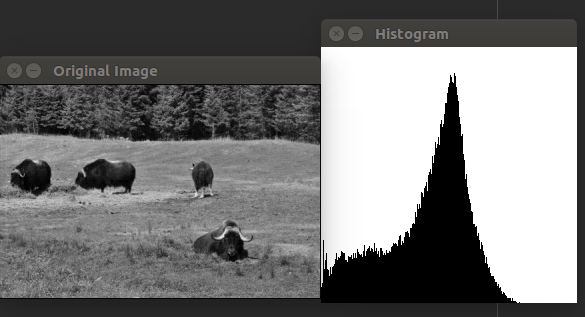##### 多通道[彩色]
###### main.cpp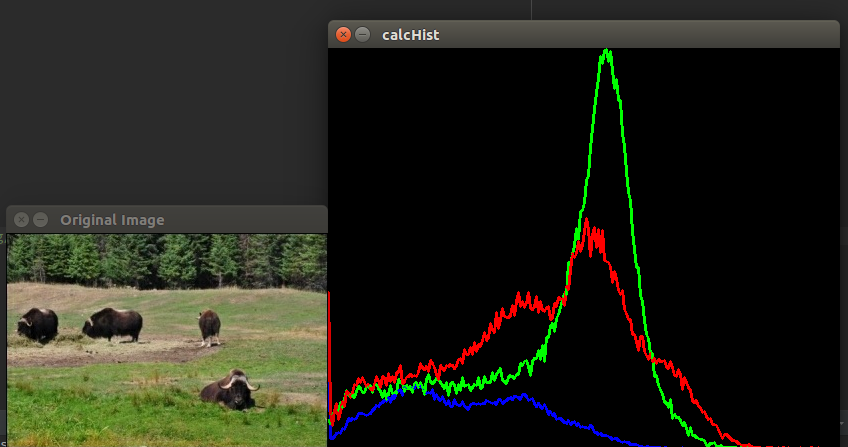##### 直方图的修正

###### 直方图的均衡化(histogram equalization)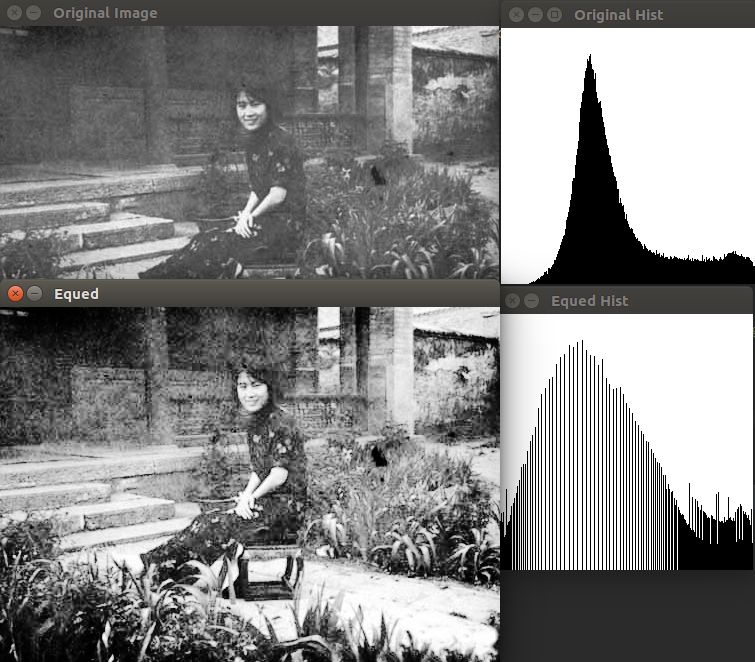###### 直方图的规定化(histogram specification/matching)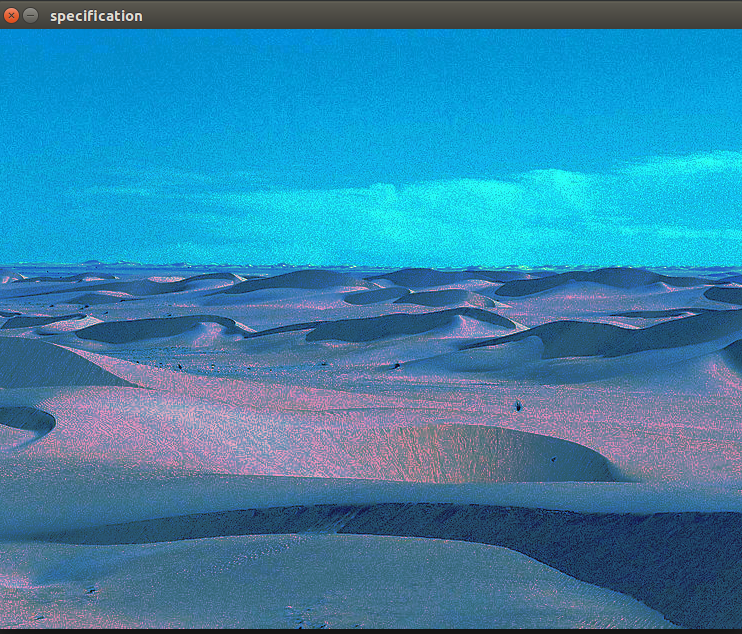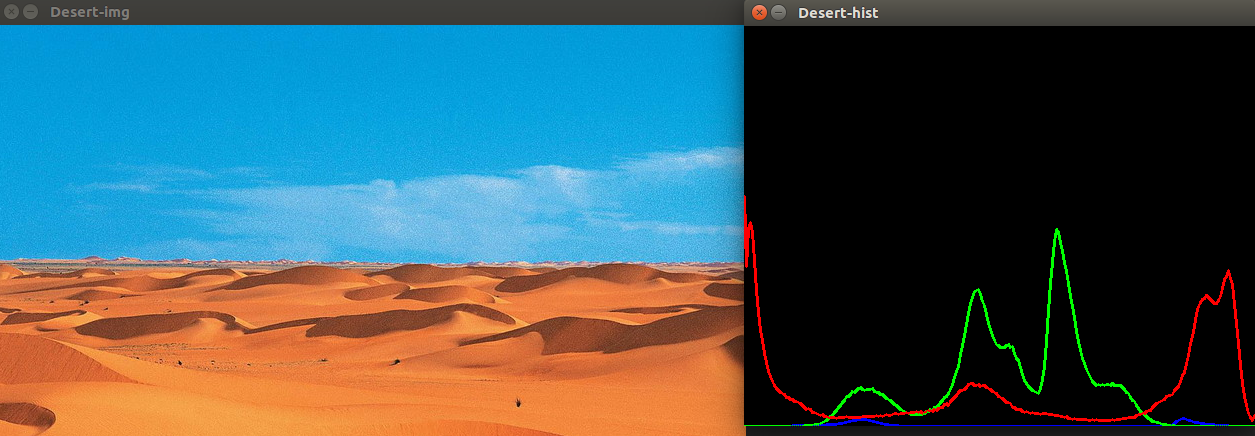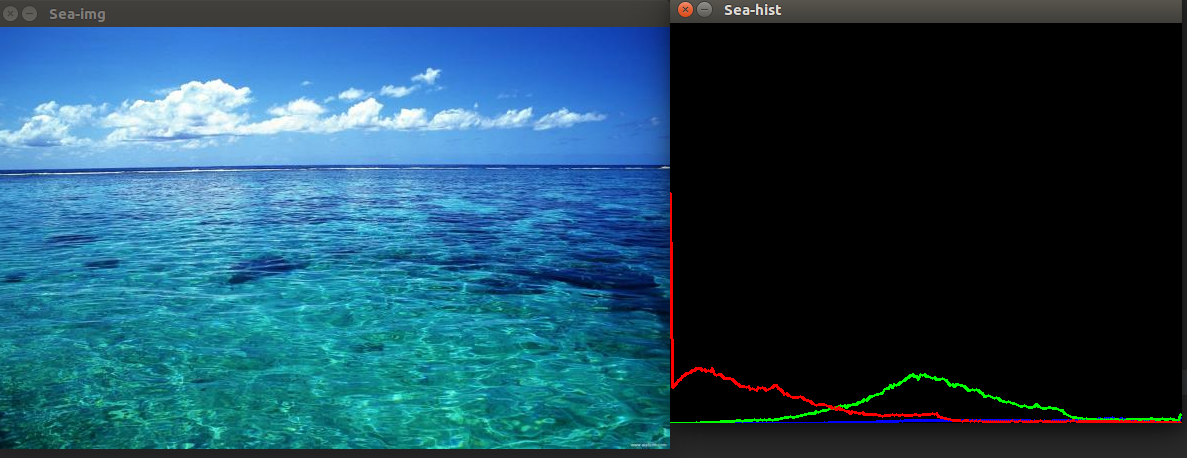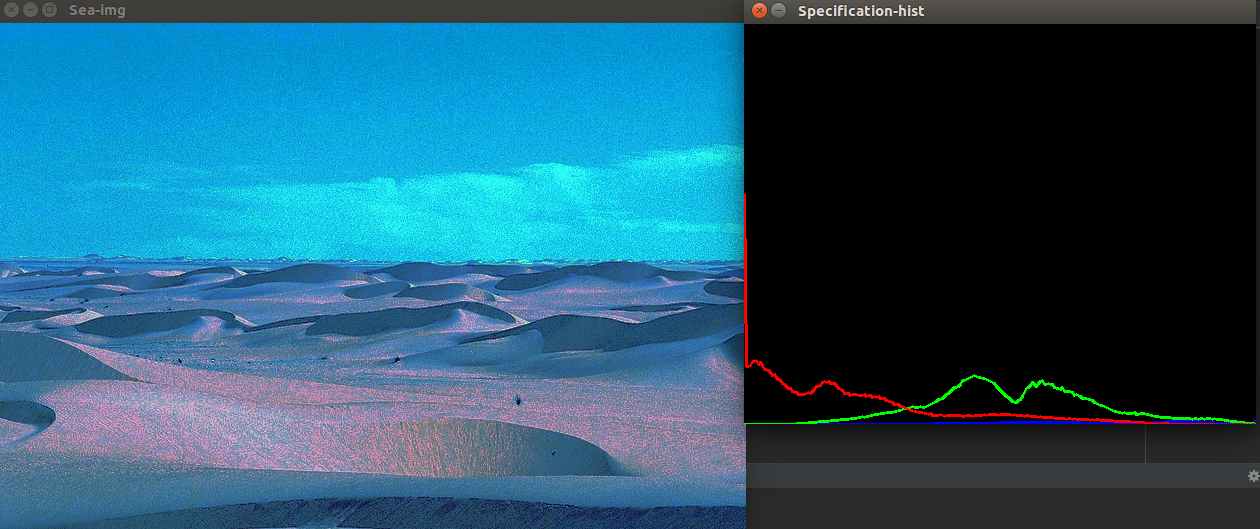#### 直方图比较Scribd代码